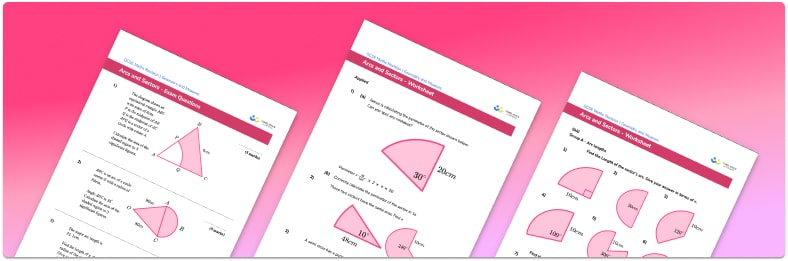# Arcs And Sectors Worksheet• Section 1 of the arcs and sectors worksheet contains 33 skills-based arcs and sectors questions, in 3 groups to support differentiation
• Section 2 contains 4 applied arcs and sectors questions with a mix of worded problems and deeper problem solving questions
• Section 3 contains 3 foundation and higher level GCSE exam questions
• Answers and a mark scheme for all questions are provided
• Questions follow variation theory with plenty of opportunities for students to work independently at their own level
• All questions created by fully qualified expert secondary maths teachers
• Suitable for GCSE maths revision for AQA, OCR and Edexcel exam boards

• This field is for validation purposes and should be left unchanged.

You can unsubscribe at any time (each email we send will contain an easy way to unsubscribe). To find out more about how we use your data, see our privacy policy.

### Arcs and sectors at a glance

An arc of a circle is a portion of the circumference of a circle. To find the length of an arc, we adapt the formula for the circumference of a circle πD, where D is the diameter of the circle. We need to divide the angle of the sector by 360, and multiply this by πD to find the length of the arc. Essentially we are finding the required proportion of the circumference of the circle.

A sector of a circle is a portion of a circle enclosed by two radii and an arc. To find the area of a sector we need to adapt the formula for the area of a circle r², where r is the radius of the circle. A simple example is calculating the area of a semicircle. Here we need to find the area of the whole circle and then divide by 2; this is because a semicircle is half of a circle. For other sectors, we need to divide the angle of the sector by 360 and then multiply this by πr² to find the area of the sector.

The algebraic formula for arc length and area of a sector frequently use Θ for the angle at the centre of the circle so it is important that students recognise this notation.

The perimeter of the sector can be found by summing the arc length and two lots of the length of the radius. As problems involving arcs and sectors use π, answers will often require rounding to a given number of decimal places or significant figures. This topic can be extended further to involve finding the area of a segment which often also requires applications of trigonometry.

Looking forward, students can then progress to additional circles, sectors and arcs worksheets and other geometry worksheets, for example an angles in polygons worksheet or area and circumference of a circle worksheet.For more teaching and learning support on Geometry our GCSE maths lessons provide step by step support for all GCSE maths concepts.

## Do you have KS4 students who need more focused attention to succeed at GCSE?There will be students in your class who require individual attention to help them succeed in their maths GCSEs. In a class of 30, it’s not always easy to provide.

Help your students feel confident with exam-style questions and the strategies they’ll need to answer them correctly with our dedicated GCSE maths revision programme.

Lessons are selected to provide support where each student needs it most, and specially-trained GCSE maths tutors adapt the pitch and pace of each lesson. This ensures a personalised revision programme that raises grades and boosts confidence.

Find out more